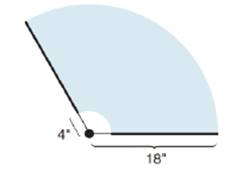Chapter 8.5, Problem 41EElementary Geometry For College St...

7th Edition
Alexander + 2 others
ISBN: 9781337614085

Solutions

Chapter
SectionElementary Geometry For College St...

7th Edition
Alexander + 2 others
ISBN: 9781337614085
Textbook Problem

In Exercises 41 and 42, round to two decimal places.A windshield wiper rotates through a 1200 angle as it cleans a windshield. From the point of rotation, the wiper blade begins at a distance of 4 in. and ends at a distance of 18 in. (The wiper blade is 14 in. in length.) Find the area cleaned by the wiper blade.To determine

To find:

The area cleaned by the wiper blade.

Explanation

Formula:

Area of sector:

If r is the radius of the circle, the area A of a sector whose arc has degree measure m is given by

A=m360×πr2

Calculation:

To find the area cleaned by wiper blade, we have to subtract the area of inner sector with radius 4 in. from the area of outer sector with radius 18 in.

Area cleaned by wiper blade =Aouter sector-Ainner sector

It is given that the wiper blade sweeps through an angle of 1200 as it cleans the windshield.

Hence the degree measure of arc is 1200.

Let’s find the area of outer sector.

From the diagram, it is clear that the radius r1 of outer sector is 18 in.

Let’s substitute the value of radius of outer sector and m=1200 to find the area of outer sector.

Asector=m360×πr12

Aouter sector=120360×π×182

Simplify:Aouter sector=13×π×324

Simplify: Aouter sector=108π in2.

Let’s substitute the value of π3.14

Aouter sector=108×3

Still sussing out bartleby?

Check out a sample textbook solution.

See a sample solution

The Solution to Your Study Problems

Bartleby provides explanations to thousands of textbook problems written by our experts, many with advanced degrees!

Get Started

In Exercises 6372, evaluate the expression. 65. 12+41612

Applied Calculus for the Managerial, Life, and Social Sciences: A Brief Approach

Compute P7,7.

Understanding Basic Statistics

Convert the expressions in Exercises 8596 radical form. 94(1x)7/3

Finite Mathematics and Applied Calculus (MindTap Course List)

If f(2) = 10 and f'(x) = x2f(x) for all x, find f"(2).

Single Variable Calculus: Early Transcendentals, Volume I

The polar form for the graph at the right is:

Study Guide for Stewart's Single Variable Calculus: Early Transcendentals, 8th